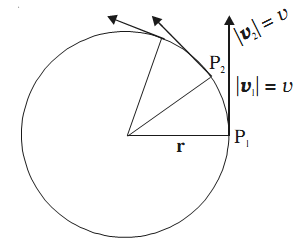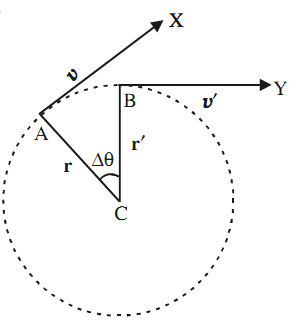# Uniform Circular Motion

The motion of a gramophone record, a grinding wheel at constant speed, the moving hands of an ordinary clock, a vehicle turning around a corner are examples of circular motion. The movement of gears, pulleys and wheels also involve circular motion. The simplest kind of circular motion is uniform circular motion.

Uniform circular motion is motion with constant speed in a circle. In uniform circular motion, the velocity vector changes continuously. This is because the direction of velocity is not constant. It goes on changing continuously as the particle travels around the circle. Because of this change in velocity, uniform circular motion is accelerated motion.The acceleration of a particle in uniform circular motion is termed as centripetal acceleration.

### Centripetal Acceleration

Consider a particle of mass m moving with a uniform speed v in a circle. Suppose at any instant its position is at A and its motion is directed along AX. After a small time ∆t, the particle reaches B and its velocity is represented by the tangent at B directed along BY.a = ∆v/∆t

a = v2/r

F = ma = mv2/r = mrω2

Some minimum centripetal force has to be applied on a body to make it move in a circular path. In the absence of such a force, the body will move in a straight line path.

The net external force directed towards the centre of the circle is called centripetal force.